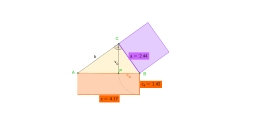# Perpendicular 32733

Calculate the right triangle ABC, the perpendicular b = 43.5 cm of the hypotenuse c = 72.9 cm. Calculate
cb, a, ca, v?

a =  58.4992 cm
c1 =  46.9432 cm
c2 =  25.9568 cm
h =  34.9069 cm

### Step-by-step explanation:Did you find an error or inaccuracy? Feel free to write us. Thank you!

Tips for related online calculators